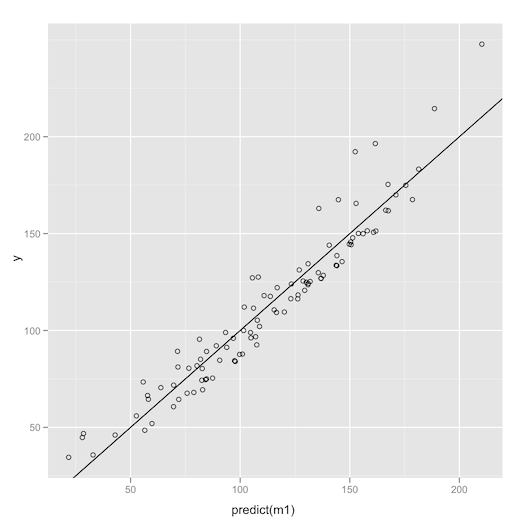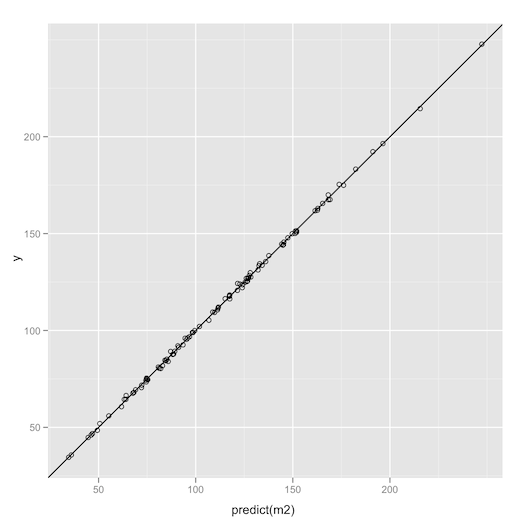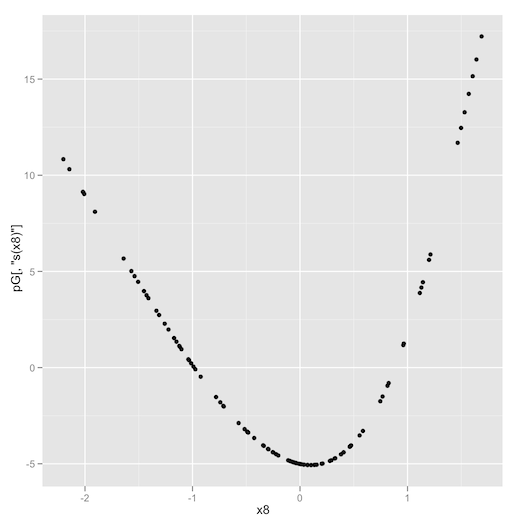# The cranky guide to trying R packages

This is a tutorial on how to try out a new package in R. The summary is: expect errors, search out errors and don’t start with the built in examples or real data.

Suppose you want to try out a novel statistical technique? A good fraction of the time R is your best bet for a first trial. Take as an example general additive models (“Generalized Additive Models,” Trevor J Hastie, Robert Tibshirani, Statistical Science (1986) vol. 1 (3) pp. 297-318); R has a package named “gam” written by Trevor Hastie himself. But, like most R packages, trying the package from the supplied documentation brings in unfamiliar data and concerns. It is best to start small and quickly test if the package itself is suitable to your needs. We give a quick outline of how to learn such a package and quickly find out if the package is for you.

To start, install and activate the package in R:

```install.packages('gam')
library(gam)
help(gam)
```

From the help we see gam fits in much the same way lm() and glm() do. So we need some data to try it out. I suggest not using the package example data or a real problem- use deliberately trivial data so you are diagnosing the new package (not diagnosing something else). I like to create a quick data frame as follows:

```d <- data.frame(
x1=rnorm(100),
x2=sample(100),
x3=0*(1:100),
x4=sample(c('a','b','c'),size=100,replace=T),
x5=as.factor(sample(c('d','e','f'),size=100,replace=T)),
x6=sample(c(F,T),size=100,replace=T),
x7=NA + 1:100,
stringsAsFactors=F)
```

Right now d is a data frame with a lot of variable types we are likely to see in practice (we have left out ordered factors):

• Nicely distributed continuous values (x1)
• Integer values (x2)
• Stuck or constant varible (x3)
• String values (x4)
• Factor values (x5)
• Logical values (x6)
• A heap of missing values (x7)

We now augment our data frame with one more input (a duplicated variable) and an output (to be predicted):

```d\$x8 = d\$x1
d\$y = rnorm(100)
+ with(d,20*exp(x1) + x2
+ 7*as.integer(as.factor(x4))
+ 9*as.integer(x5) + 10*as.integer(x6))
```

This is our simple test data set. Our standard method of fitting a linear model for y (before trying the gam package) is either to (falsely) assume x1’s contribution is linear or to (amazingly) guess the exact transformation required is exp(x1).

In the first case the model looks like this (using the ggplot2 package):

(As a side note- statisticians usually cluck if you ask for a graph showing “truth” as a function of “prediction” (or y ~ f(x)). They say you should be looking at residuals (or (y-f(x) ~ f(x)) instead (and usually only supply functions that produce residual plots). But in their own publications, such as the paper we started with, when they want to make a point: they actually plot truth as a function of prediction.)

```m1 <- lm(y ~ x1 + x2 + x3 + x4 + x5 + x6 + x8, data=d)
ggplot(d,aes(predict(m1),y)) + geom_point(shape=1)
+ geom_abline(slope=1) + opts(aspect.ratio=1)
```And in the second case the model looks like this:

```m2 <- lm(y ~ exp(x1) + x2 + x3 + x4 + x5 + x6 + x8, data=d)
ggplot(d,aes(predict(m2),y)) + geom_point(shape=1)
+ geom_abline(slope=1) + opts(aspect.ratio=1)
```(In both cases we had to leave out x7, which was all NAs.) Notice how knowing the functional form of x1 moves us from a good fit to an extraordinary fit. We would like to automatically learn the form of x1’s contribution from data and get this better fit automatically. This is in fact the point of the gam package. To perform the gam fit we add the
smoothing symbol s() to each variable we want to try and learn the possibly non-linear shape of contribution of.

```mG <- gam(y ~ s(x1) + s(x2) + x3 + x4 + x5 + x6 + s(x8), data=d)
ggplot(d,aes(predict(mG),y)) + geom_point(shape=1)
+ geom_abline(slope=1) + opts(aspect.ratio=1)
```

We could not add the s() symbol to any of the un-ordered factors, strings, logicals, the NAs or constant columns. Except for that the gam package is performing as robustly as the built in lm() package and produces a fit essentially as good as knowing the shape of x1’s contribution ahead of time:That is the good part. But it just wouldn’t be an R-package without some bad parts.

The first problem is: at first glance the gam package plot() appears as if it is broken (or at least not a compatible extension of plot.lm or plot.glm, classes even though gam claims to extend both of those classes). Traditionally when you call plot() on a model it steps you through a bunch of arcane graphs that statisticians swear are more important than examining the fit directly. But plot(mG) seems to step through all of a different family of graphs without waiting for user input, and we are left only with the graph that presumably shows the shape adjustment of variable x8. Only if you anticipate plot() is different for gam than for other models (or dump the function code for plot.gam) do you learn you need to add the argument ask=T. plot(mG,ask=T) enters an interactive mode where you can see the inferred shape of each variable. That is, there is an argument that defaults to a value you don’t want (or as we say in industry: “you forgot to set the don’t lose flag.”). gam is actually a very high quality package, but these sort of “poison defaults” are something you have to be in the habit of looking out for in R.

The second problem is intrinsic to the method: we are not guaranteed that s(x1) or s(x8) either look much like exp(x1). It is only guaranteed that some linear of them does (as that was how they were used in the model). We can get direct access to the learned reshapings by calling predict() to ask for term contributions and see how the model is linear in the transformed coordinates (essentially with all coefficients 1).

```pG = predict(mG,type='terms')
summary(lm(d\$y ~ pG))

Call:
lm(formula = d\$y ~ pG)
Residuals:
Min      1Q  Median      3Q     Max
-3.5690 -0.7121 -0.0288  0.6924  5.3883
Coefficients: (1 not defined because of singularities)
Estimate Std. Error t value Pr(>|t|)
(Intercept) 1.125e+02  1.363e-01  824.92   <2e-16 ***
pGs(x1)     9.994e-01  6.486e-03  154.09   <2e-16 ***
pGs(x2)     1.002e+00  4.905e-03  204.19   <2e-16 ***
pGx3               NA         NA      NA       NA
pGx4        9.973e-01  2.548e-02   39.14   <2e-16 ***
pGx5        1.004e+00  1.926e-02   52.10   <2e-16 ***
pGx6        9.967e-01  2.796e-02   35.64   <2e-16 ***
pGs(x8)     1.057e+00  2.520e-02   41.96   <2e-16 ***
---
Signif. codes:  0 ‘***’ 0.001 ‘**’ 0.01 ‘*’ 0.05 ‘.’ 0.1 ‘ ’ 1
```

We can examine the transforms (like s(x8) = f(x8)) by plotting:

```ggplot(d) + geom_point(aes(x8,pG[,'s(x8)']))
```Even though s(x8) has a funny shape it plus s(x1) are an excellent approximation of exp(x1) (with commensurate magnitudes):

```> lm <- lm(exp(d\$x1)~pG[,'s(x1)']+pG[,'s(x8)'])
> summary(lm)

Call:
lm(formula = exp(d\$x1) ~ pG[, "s(x1)"] + pG[, "s(x8)"])
Residuals:
Min        1Q    Median        3Q       Max
-0.118086 -0.014125  0.002899  0.023389  0.217152
Coefficients:
Estimate Std. Error t value Pr(>|t|)
(Intercept)   1.2536112  0.0046410  270.12   <2e-16 ***
pG[, "s(x1)"] 0.0500079  0.0002126  235.25   <2e-16 ***
pG[, "s(x8)"] 0.0534411  0.0008349   64.01   <2e-16 ***
---
Signif. codes:  0 ‘***’ 0.001 ‘**’ 0.01 ‘*’ 0.05 ‘.’ 0.1 ‘ ’ 1
Residual standard error: 0.04641 on 97 degrees of freedom
Multiple R-squared: 0.9987,	Adjusted R-squared: 0.9986
F-statistic: 3.588e+04 on 2 and 97 DF,  p-value: < 2.2e-16
```

The functions do have different variances (s(x1) is doing most of the work):

```> var(pG[,'s(x1)'])
 514.8578
> var(pG[,'s(x8)'])
 33.37209
```

Yet the coefficients of mG seem to be gibberish (notice the 0 on s(x8)):

```> mG\$coefficients
(Intercept)       s(x1)       s(x2)          x3         x4b         x4c         x5e
45.580747   22.749160    1.007585    0.000000    7.048177   13.346365    8.740460
x5f      x6TRUE       s(x8)
18.003981   10.102217    0.000000
```

So by poking around we have learned not to look at this slot of the returned model (and it is much cheaper to learn this through this cranky poking around on a trivial example than to learn it while trying to analyze real data or blundering through R’s overly operational documentation).

The third (and last) problem is one of attitude (and one of the barriers to learning statistics). There is not a lot of support for exporting the derived gam smoothers (the transforms on the input variables) from R. The original paper suggests that you should think of the non-parametric smoothers as graphs and user linear interpolation between your data points. You can do this by calling “predict(mG,type=’terms’)” as we did above. Or you can try to switch to parametric splines and then run into the same problem that the splines package is not really export friendly. Or you can ask around. The R community is generally quite bright and friendly- but every once in a while you get a whiff of statistics territorialism (or perhaps a defensiveness, where if you are correct but not fully general you fear you will look shallow). Sensible requirements, like wanting to export usable model parameters to another system, are considered naive. A favorite example of mine: in this help thread somebody who is asking how to configure and export explicit spline transforms to meet an external requirement to get their paper published is advised: “I think that the referee is being unreasonable here. There are many perfectly respectable ways of estimating GAMs for which no explicit expression for the estimated smooth terms is available (See Hastie and Tibshirani’s GAM book).” And then offered additional references to help educate the referee (instead of recognizing that an explicit sharable solution in hand could, in some cases, be more useful than a maximally general solution that can’t be communicated succinctly). It is like lecturing a drowning man on how important water is to fish.

In summary- never first try a new R package on real data. R packages are often realization of very deep concepts from the literature that bring in their own terminology, trade-offs and attitudes. You need time to absorb these things in isolation. Expect and forgive non-essential errors (many important and valuable packages have them). Approach new packages with a cranky inquisitiveness about the package, otherwise you may actually fall into a non-productive state of frustration.

Also, gam methods are amazing.

(note: we also recommend trying the mgcv package for gam modeling. It represents a different set of tradeoffs.)

Categories: Opinion Pragmatic Data Science Tutorials### jmount

Data Scientist and trainer at Win Vector LLC. One of the authors of Practical Data Science with R.